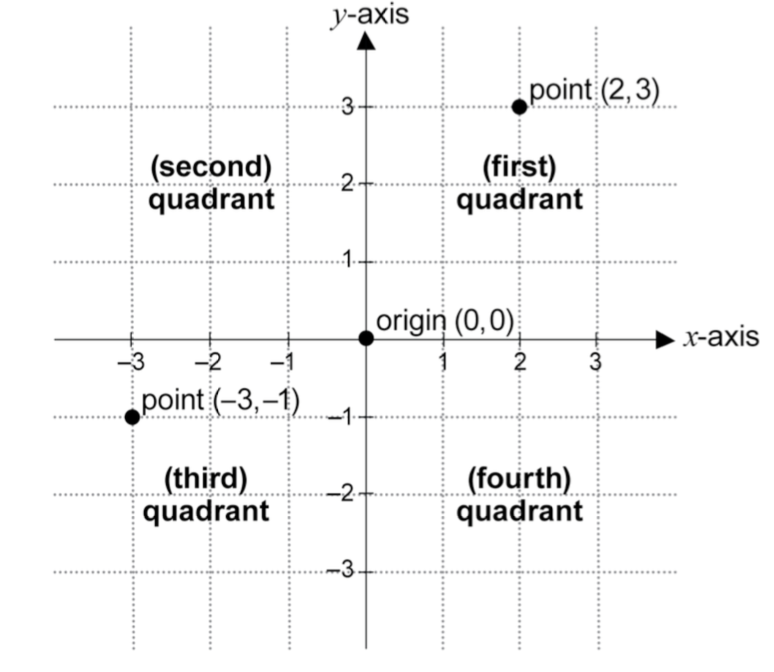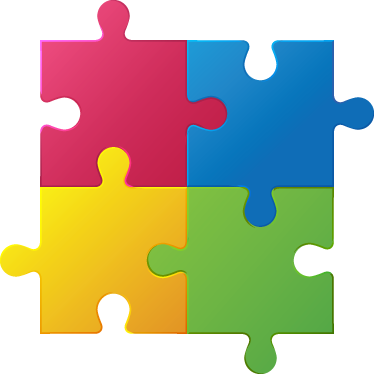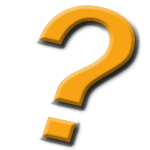# Year Five - Exploring the Coordinate Plane

In this Big Idea students explore the concepts of number, position and patterns using the cartesian plane. Students conclude their inquiry be exploring patterns within the multiplication grid.

### Understanding Goals:

Students will understand:

• that the coordinate plane allows two different dimensions of an object to be shown with one small point.
• that many mathematical ideas can be shared on a coordinate plane.
• that points plotted on the coordinate plane have meaning.
• that patterns exist in our multiplication table and why this is so.

### Background:

The Cartesian plane (commonly referred to as the 'number plane') is named after the French philosopher and mathematician René Descartes (1596–1650), who was one of the first to develop analytical geometry on the number plane. On the number plane, the 'coordinates of a point' refers to the ordered pair describing the horizontal position x first, followed by the vertical position y.

The Cartesian plane is applied in real-world contexts, eg when determining the incline (slope) of a road between two points.

Note - Students return to an exploration of the coordinate plane in Year Six when they investigate "Expanding the Number Line" and negative integers. In this Year Five unit students are not required to develop an understanding of negative integers.

### Core Content from the Syllabus:

Working Mathematically

Patterns & Algebra

• recognise that the number plane (Cartesian plane) is a visual way of describing location on a grid
• recognise that the number plane consists of a horizontal axis (x-axis) and a vertical axis (y-axis), creating four quadrants
• recognise that the horizontal axis and the vertical axis meet at right angles (Reasoning)
• identify the point of intersection of the two axes as the origin, having coordinates (0, 0)
• plot and label points, given coordinates, in all four quadrants of the number plane ! plot a sequence of coordinates to create a picture (Communicating)
• identify and record the coordinates of given points in all four quadrants of the number plane
• recognise that the order of coordinates is important when locating points on the number plane, eg (2, 3) is a location different from (3, 2) (Communicating)Position

• Use a grid-reference system to describe locations
• describe particular locations on grid-referenced maps, including maps with a legend, eg 'The post office is at E4'

Two-Dimensional Space

• identify and draw regular and irregular two-dimensional shapes from descriptions of their side and angle properties

Patterns & Algebra

In Stage 3, students should be encouraged to use their own words to describe number patterns. Patterns can usually be described in more than one way and it is important for students to hear how other students describe the same pattern. Students' descriptions of number patterns can then become more sophisticated as they experience a variety of ways of describing the same pattern.

### Language:

• number plane (Cartesian plane), horizontal axis (x-axis), vertical axis (y-axis), axes, quadrant, intersect, point of intersection, right angles, origin, coordinates, point, plot.
• pattern, increase, decrease, number sentence, number line

## Mindset Mathematics Learning Activities

###Visualise

Students explore the meaning of the coordinate plane by interpreting and creating four-quadrant graphs, using qualitative comparisons instead of values on the axes. - See page 118

Questions for reflection:

• What does a four-quadrant graph enable you to communicate? What can you learn from this kind of graph?

###Play

Students play with describing and locating points— and the shapes they make— in the first quadrant of the coordinate plane in a game called Ship Shape. - See page 124

Questions for reflection:

• What strategies did you come up with to help you find shapes?
• What was challenging about finding the shapes on the coordinate plane?
• How did you record the results of your guesses to keep track of where shapes might or might not be?

###Investigate

Students explore patterns in the multiplication table and then investigate what happens when they graph those patterns on the coordinate plane. - See page 133

Questions for reflection:

• What ways did you come up with for showing the multiplication patterns on the coordinate plane?
• What does the coordinate plane enable us to see? What do you notice that you hadn't before?
• What other repeating values did you locate? How were their patterns similar to or different from the ones we had already seen?